# GloVe 50-Dimensional Word Vectors Trained onWikipedia and Gigaword 5 Data

Represent words as vectors

Released in 2014 by the computer science department at Stanford University, this representation is trained using an original method called Global Vectors (GloVe). It encodes 400,000 tokens as unique vectors, with all tokens outside the vocabulary encoded as the zero-vector. Token case is ignored.

Number of layers: 1 | Parameter count: 20,000,050 | Trained size: 83 MB |

## Examples

### Resource retrieval

Get the pre-trained net:

 In:=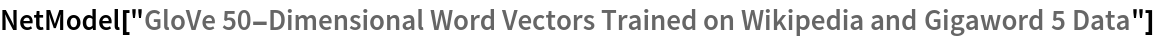Out=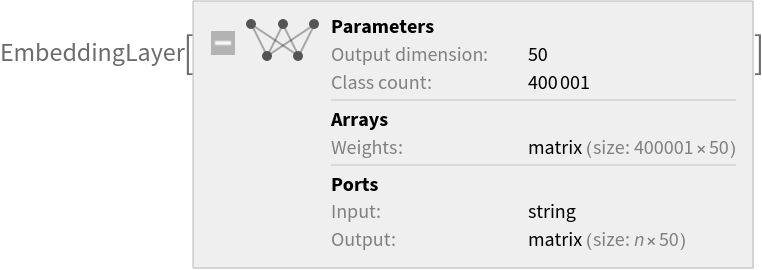### Basic usage

Use the net to obtain a list of word vectors:

 In:=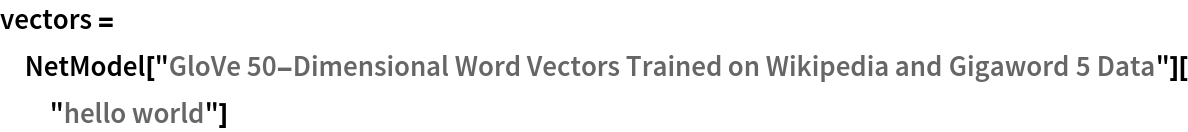Out=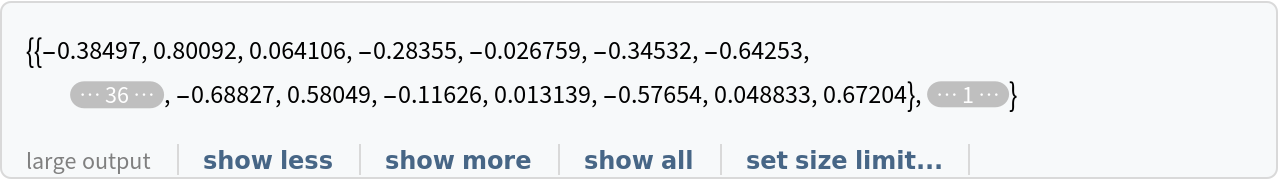Obtain the dimensions of the vectors:

 In:=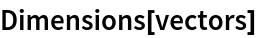Out=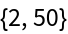Use the embedding layer inside a NetChain:

 In:=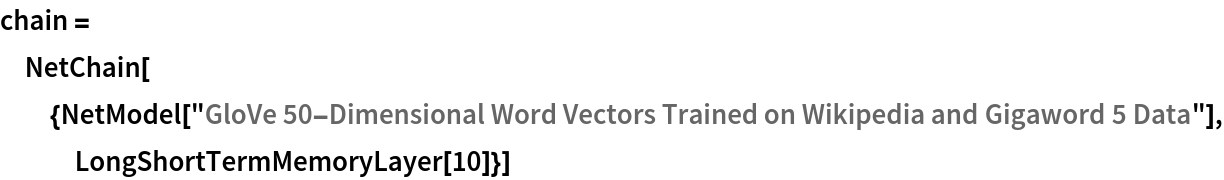Out=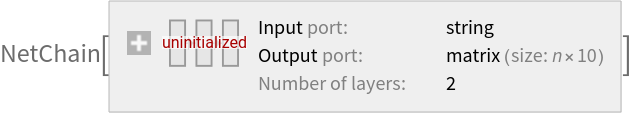### Feature visualization

Create two lists of related words:

 In:=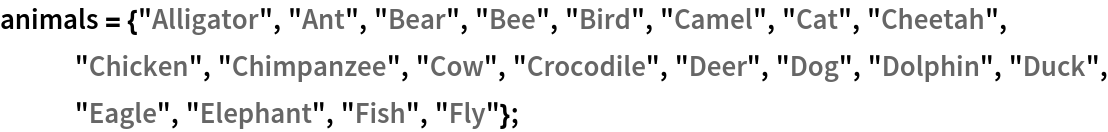In:=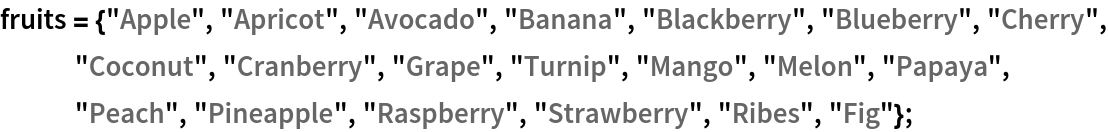Visualize relationships between the words using the net as a feature extractor:

 In:=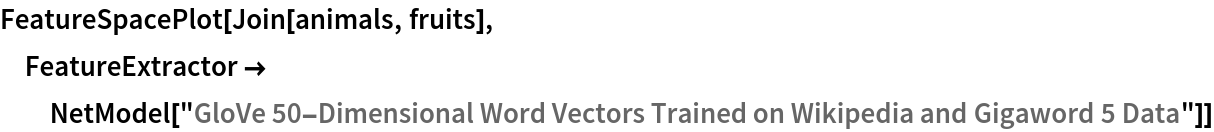Out=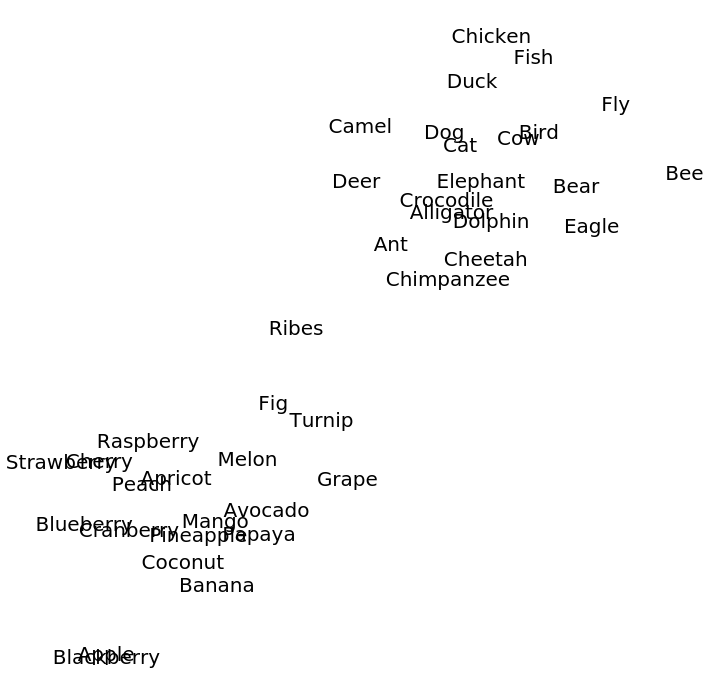### Word analogies

Get the pre-trained net:

 In:=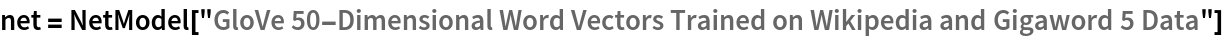Out=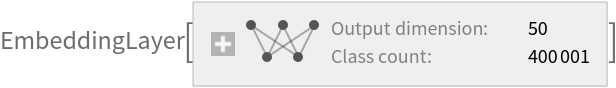Get a list of words:

 In:=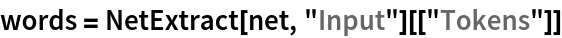Out=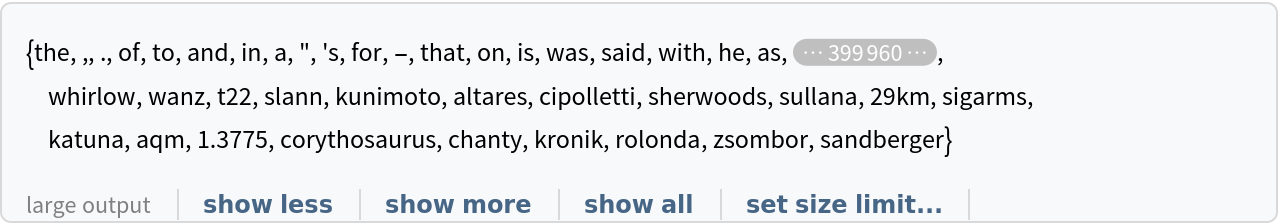Obtain the vectors:

 In:=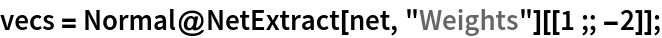Create an association whose keys are words and whose values are vectors:

 In:=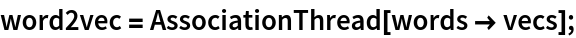Find the eight nearest words to "king":

 In:=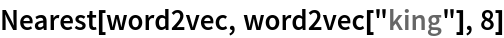Out=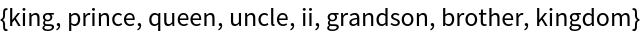Man is to king as woman is to:

 In:=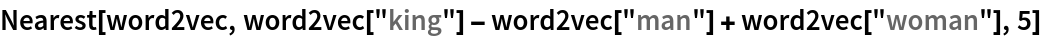Out=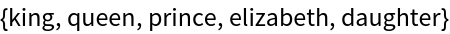France is to Paris as Germany is to:

 In:=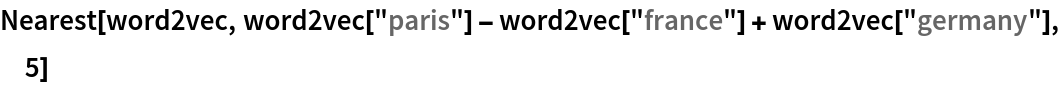Out=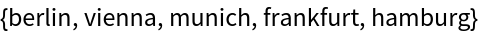### Net information

Inspect the number of parameters of all arrays in the net:

 In:=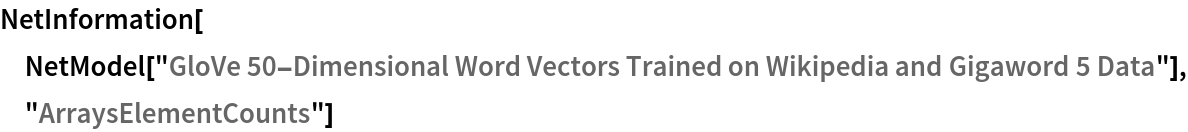Out=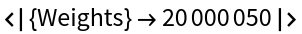Obtain the total number of parameters:

 In:=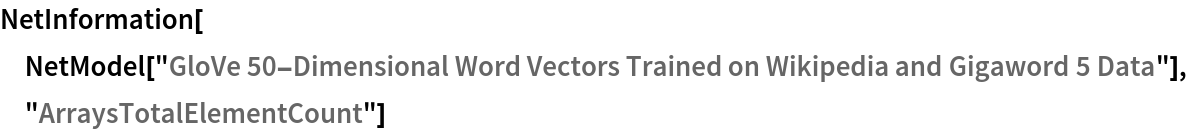Out=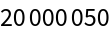Obtain the layer type counts:

 In:=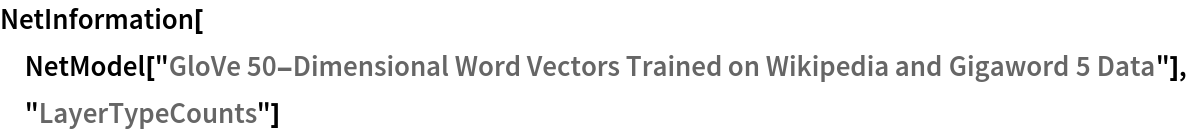Out=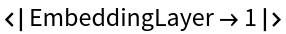### Export to MXNet

Export the net into a format that can be opened in MXNet:

 In:=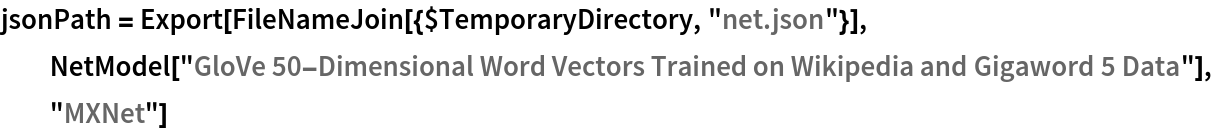Out=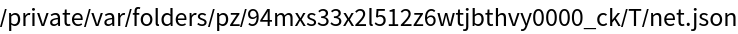Export also creates a net.params file containing parameters:

 In:=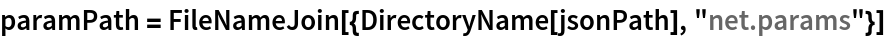Out=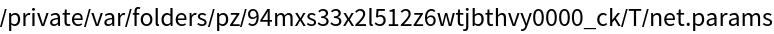Get the size of the parameter file:

 In:=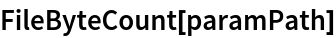Out=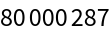The size is similar to the byte count of the resource object:

 In:=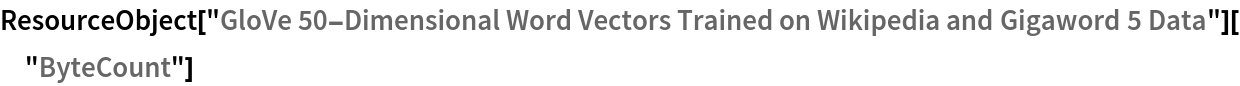Out=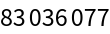Represent the MXNet net as a graph:

 In:=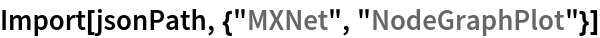Out=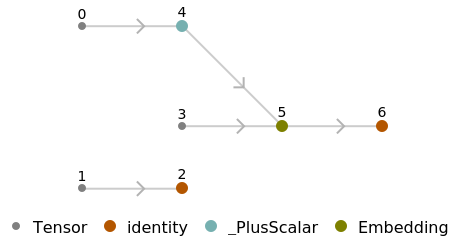## Requirements

Wolfram Language 11.1 (March 2017) or above

## Reference

• J. Pennington, R. Socher, C. D. Manning, "GloVe: Global Vectors for Word Representation," Empirical Methods in Natural Language Processing (EMNLP), 1,532-1,543 (2014)
• Available from: http://nlp.stanford.edu/projects/glove